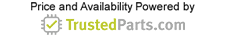Industrial Electronics

# Wolfspeed SiC MOSFET-Based, Bidirectional, Three-Phase AC/DC Converters

23 February 2018

Grid-connected, three-phase AC/DC (or DC/AC) power conversion is required in a wide range of industrial applications — from power electronic interfaces of renewable energy systems (solar, wind and batteries), industrial and mass transport vehicle charging, to regenerative motor drives (elevators, mills, etc.). A practical example of such a three-phase, grid-tied AC-DC system would be fast charging stations for electric vehicles. Figure 1 shows a functional block diagram of a three-phase fast off-board charger for battery electric vehicles (BEVs). With bidirectional functionality of the converter, it is possible to deliver power from vehicle to grid (V2G).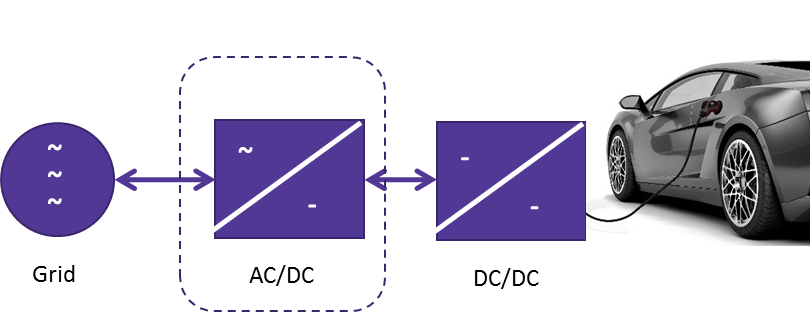Figure 1. Functional block diagram of three-phase AC/DC system.

For bidirectional applications, two-level topologies using 1200-volt insulated-gate bipolar transistors (IGBTs) or three-level topologies using SJ FETs or fast 650-volt IGBTs are typically used. Two-level topologies with 1200-volt IGBTs offer simplicity, low semiconductor cost and high-power capability (greater than 20 kilowatts), but switching frequency is limited to less than 20 kilohertz — yielding systems with lower power density, lower efficiency and expensive inductors. Multilevel topologies such as the neutral point clamped (NPC) rectifier offer higher power density and efficiency (lower switching losses) at the expense of higher circuit complexity and cost. Figure 2 shows the two-level IGBT and three-level NPC rectifier topologies.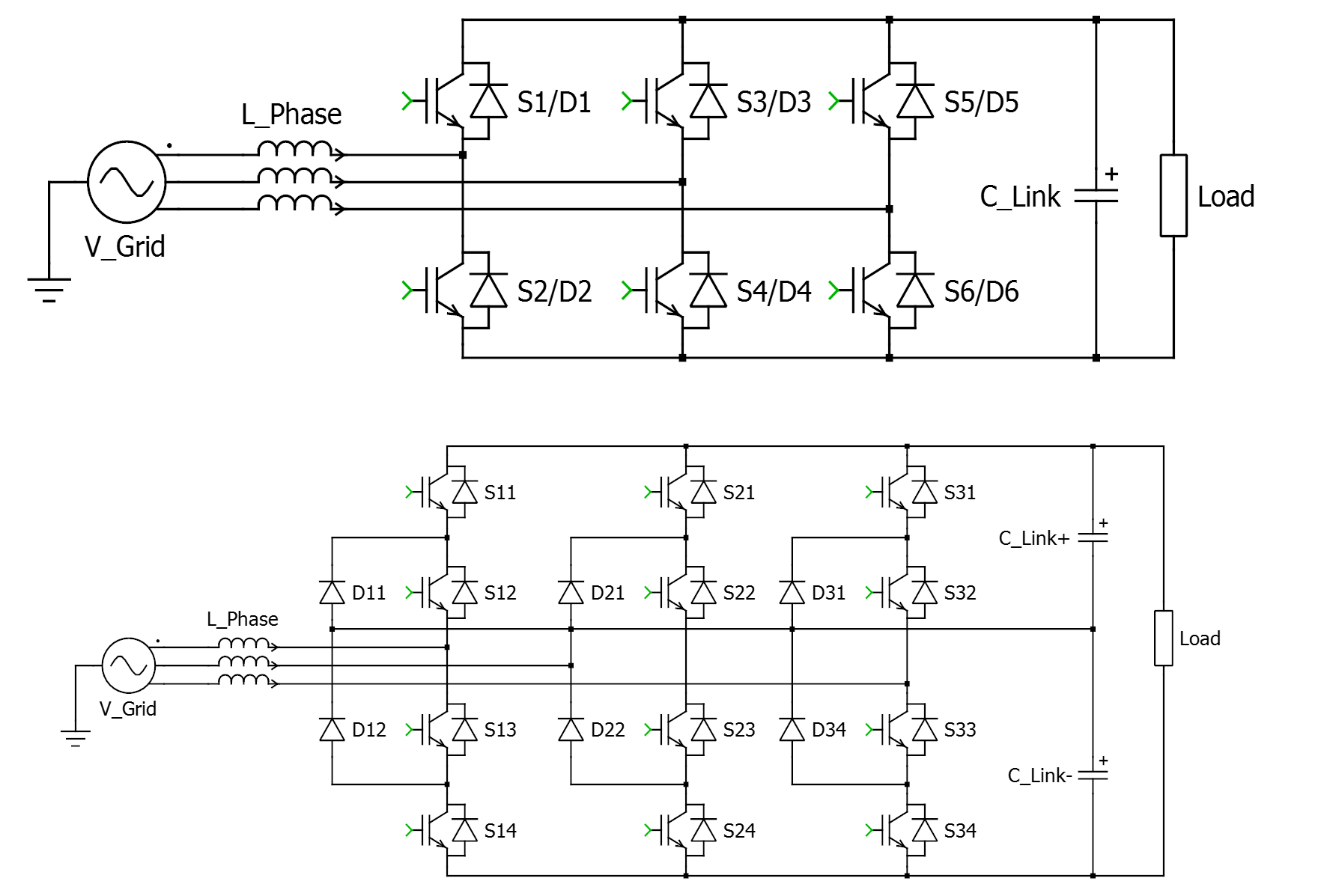Figure 2. Two-level six-switch IGBT rectifier (top) and three-level NPC rectifier (bottom).

An alternative approach, utilizing silicon carbide (SiC) MOSFETs drastically reduces switching losses compared to 1200-volt IGBTs and significantly extends the usable switching frequency range of the two-level, six-switch converter, while maintaining higher full-load and part-load efficiency. With SiC MOSFETs power density of the system is significantly increased by shrinking the size of magnetic components and reducing the size of the heatsink. Additionally, when utilizing SiC MOSFETs, the device’s body diode can be used as the anti-parallel diode, reducing circuit complexity and cost. Figure 3 shows the two-level SiC MOSFET converter power stage.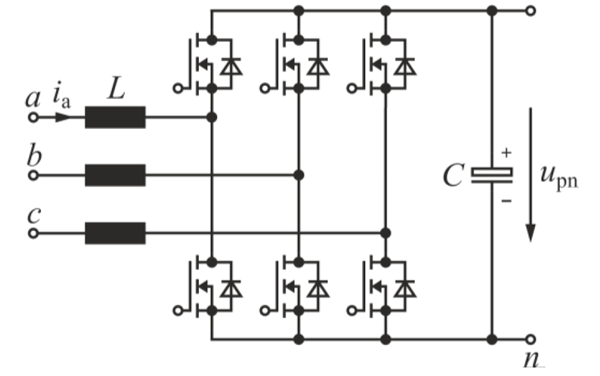Figure 3. Two-level SiC MOSFET converter.

This article showcases a cost-effective, highly efficient design alternative for an industrial PFC application with the entire design based on SiC power MOSFET devices that are fully-qualified and in volume production. Challenging the traditional 1200-volt Si-IGBT two-level system, this design meets the same system-level specifications as presented in Table I. The proposed two-level SiC system follows the power topology in Figure 3 and is compared to a six-switch silicon (Si) IGBT system in Figure 2 (top).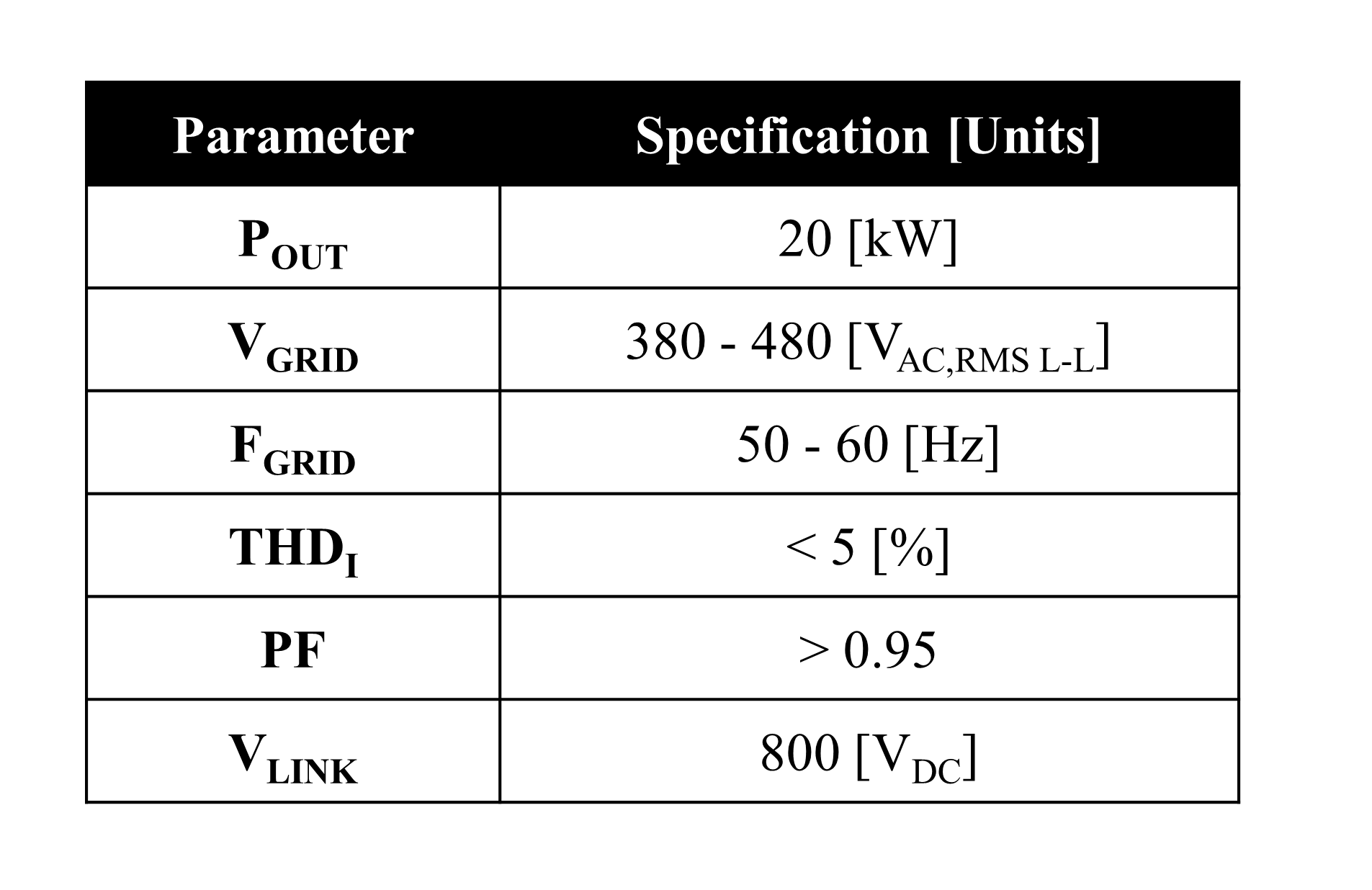Tabel 1. PFC system specifications

System Comparison of SiC MOSFET and Si-IGBT Converter

To meet the system-level specifications presented in Table I, dimensioning semiconductors for the two-level, six-switch SiC system was performed using standard three-phase, two-level voltage source inverter design equations.

For this power level, a new 1000 volt/65 mΩ SiC MOSFET was selected. This part delivers extremely low switching losses thanks to a four-lead TO-247 package with dedicated Kelvin source connection. In addition, the optimized 1000-volt blocking and rugged body diode capability allows for minimum die cost while supporting up to 800 volts direct current (VDC) link operation. Figure 4 shows the device switching loss behavior as a function of drain current. Figure 5 shows the RDSON behavior versus temperature.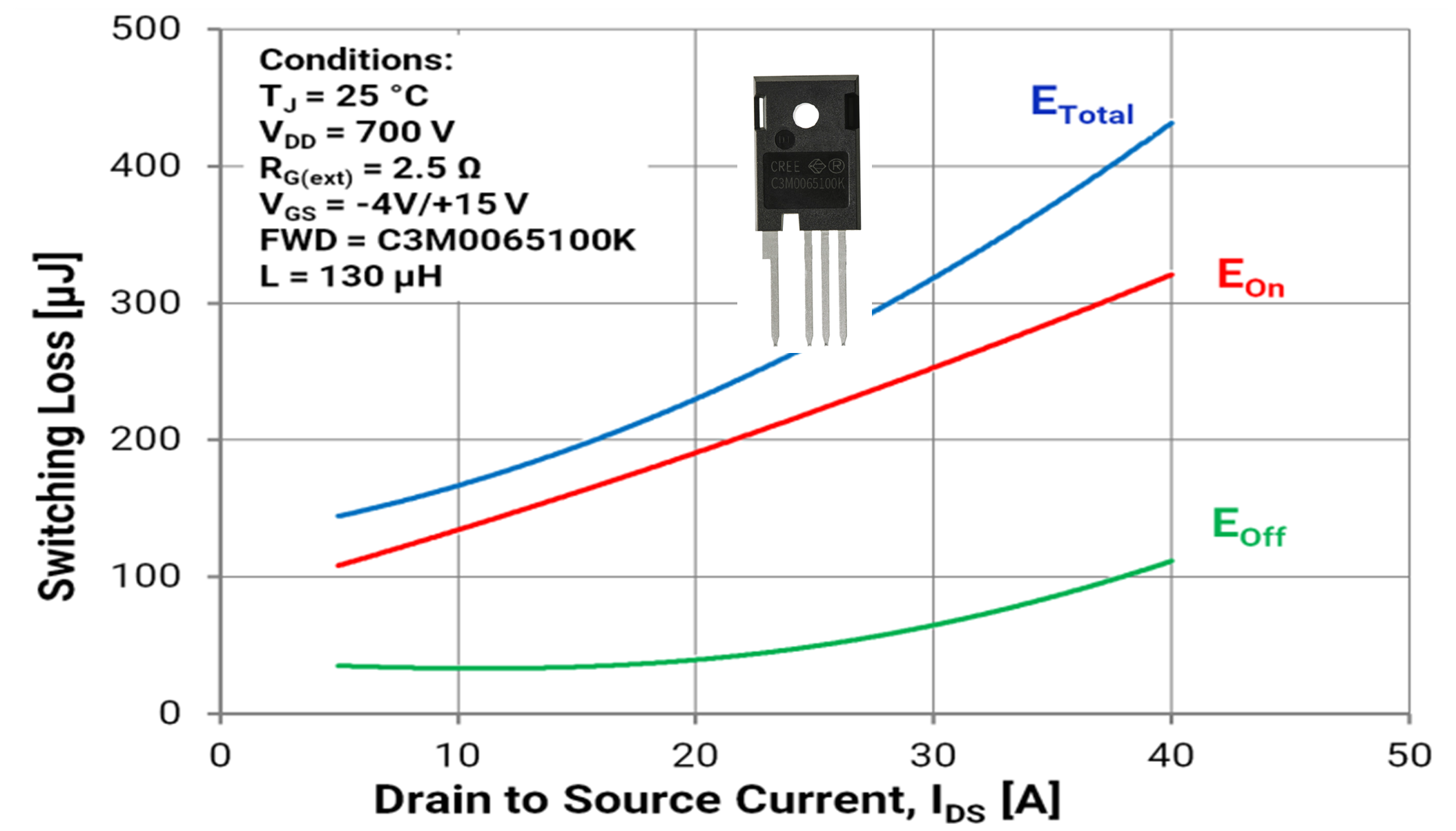Figure 4. Switching loss as a funcion of drain current for new 1000 volts/65 meters-squared SiC MOSFET.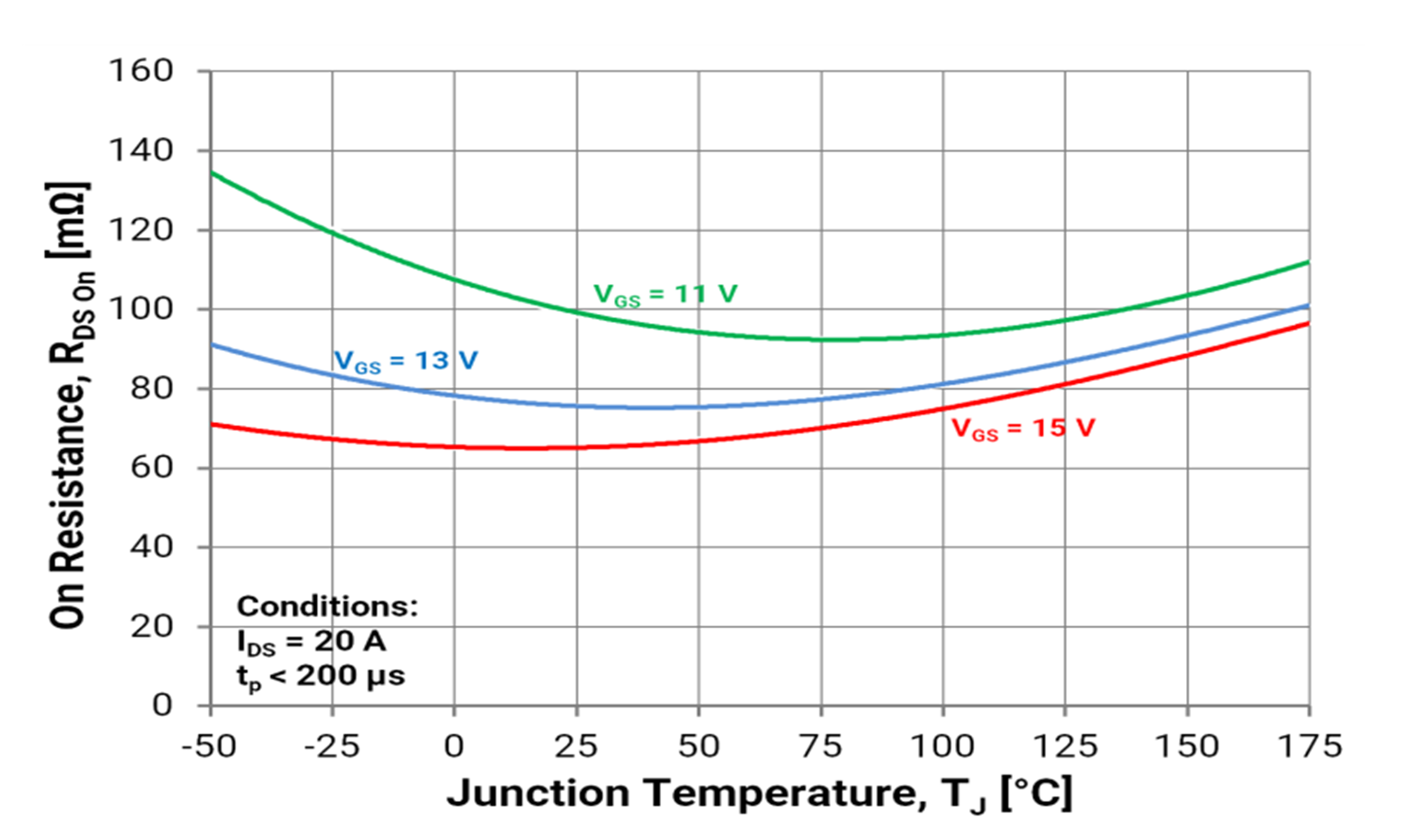Figure 5. RDSON vs. temperature for new 1000 volts/65 meters-squared SiC MOSFET.

Compared with a similar current-rated 1200-volt, 50 A Si-IGBT (IGW25N120H3), the switching loss of the IGBT is seven times higher than a SiC MOSFET (based on the datasheet specifications). This is due to the high turn-off losses found in IGBTs.

Using the device static and dynamic characteristics, the system semiconductor losses can be calculated through simulation. Figure 6 summarizes the total losses assuming a constant device junction temperature of 110 degrees Celsius. First, the SiC MOSFET and Si IGBT were simulated operating at same switching frequency, 48 kHz. At 48 kHz, the IGBT losses are approximately 900 watts, dominated mainly by switching losses. For IGBT, the frequency had to reduce to 16 kHz in order to get to acceptable total losses.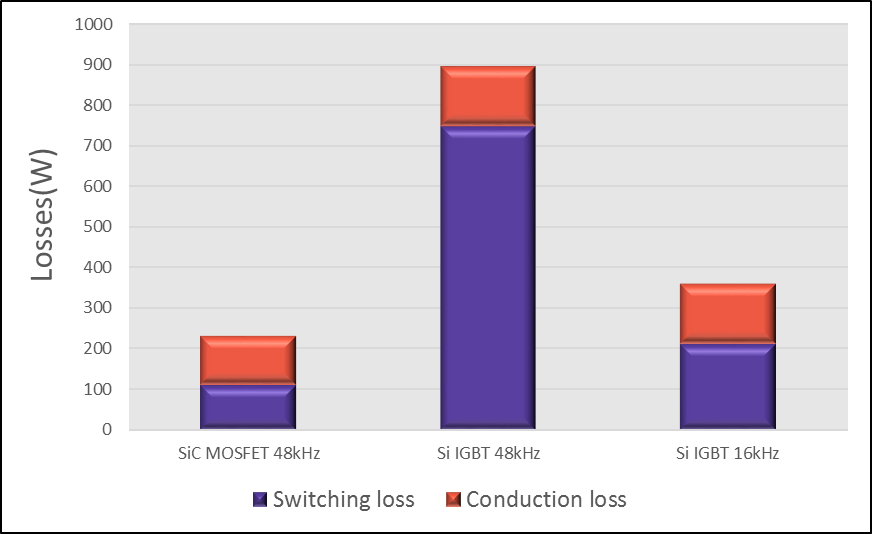Figure 6. Power loss comparison with SiC MOSFET and Si IGBT (Conditions: VGRID = 380 V, VLINK = 800 V, POUT = 20 kW assuming a constant Tj = 110° C).

In order to achieve the same ripple current, with 16-kHz switching frequency, the inductor has to be 1.2 mH. If an amorphous core is used, AMCC-50 is needed for 48 kHz and AMCC-200 for 16 kHz. The cost comparison and cost is listed in Figure 7.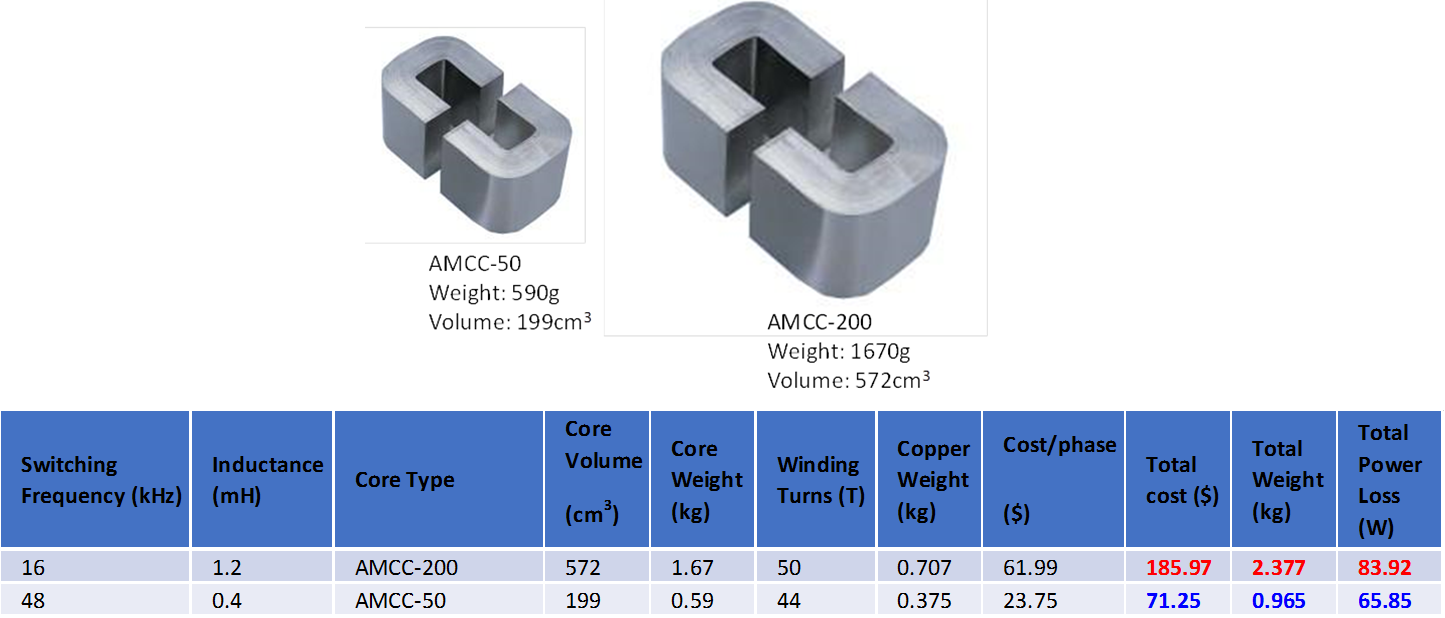Figure 7. Comparison of AMCC-50 and AMCC-200 core.

The benefit of the higher switching frequency is summarized in the table above. Due to the higher switching frequency made possible with SiC MOSFETs, the size and cost of the inductor is reduced dramatically. The volume is reduced 300 percent while the cost and phase is reduced 250 percent.

A second important difference between the proposed two-level, six-switch SiC PFC and the Si IGBT is cooling requirements for the semiconductors. The proposed two-level six-switch SiC PFC needs to dissipate a minimum power of 230 watts while the Si IGBT requirement is 360 watts. Assuming a typical extruded aluminum heatsink (Aavid Thermalloy 82160) with a 400 LFM airflow, the volume reduction from 3.9 dm3 for the Si-IGBT system to a volume of 1.7 dm3 for the SiC system is possible. Not only is this a significant size and weight reduction, but it will also bring down the cost.

Hardware Implementation and Test Results

Figure 8 shows photographs of the 20 kW, two-level SiC hardware prototype. The power stage is composed of two paralleled 1,000- volt 65 mΩ SiC MOSFETs per switch position. The devices are packaged on TO-247-4L discrete packages with a dedicated-source Kelvin connection. The devices offer a cost-optimized solution by eliminating the needed for anti-parallel Schottky diodes. This also simplifies power PCB layout and heatsink mounting.

Figure 9 shows the waveforms of input voltage and input current for Phase A under full power conditions at FSW = 48 Hz. The figure shows the alignment of voltage and current with minimal distortion, which proves two level converter works very well to achieve power factor correction.

Figure 10 summarizes the system efficiency and THD. The efficiency curves demonstrate the effectiveness of low switching loss of SiC MOSFET.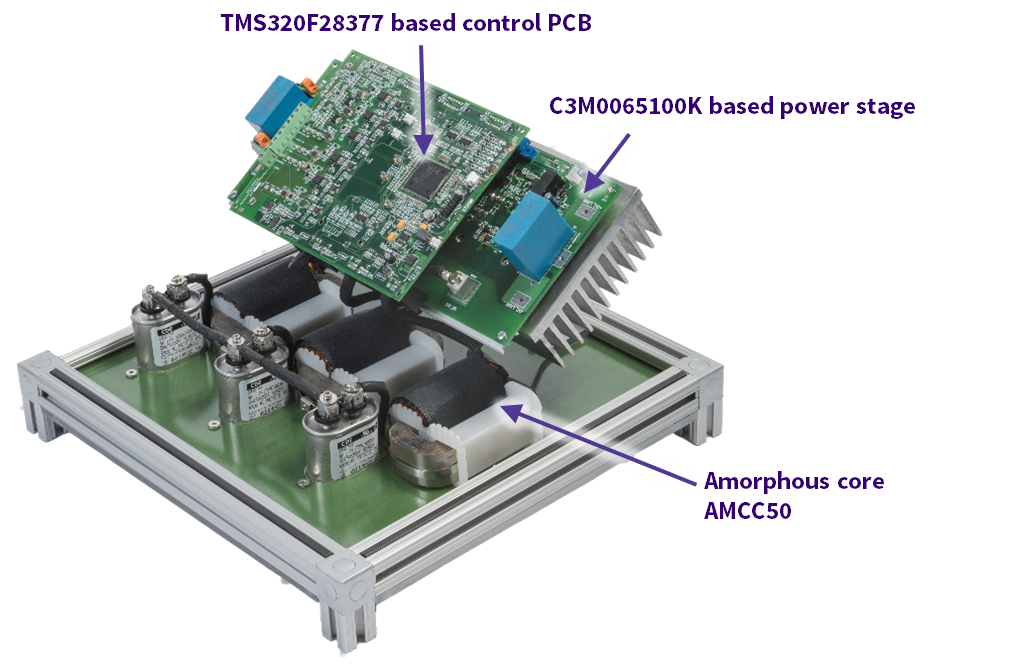Figure 8. Harware of 20 kW, three phase, bidirectional converter with 1000 volts/65 m? SiC MOSFET.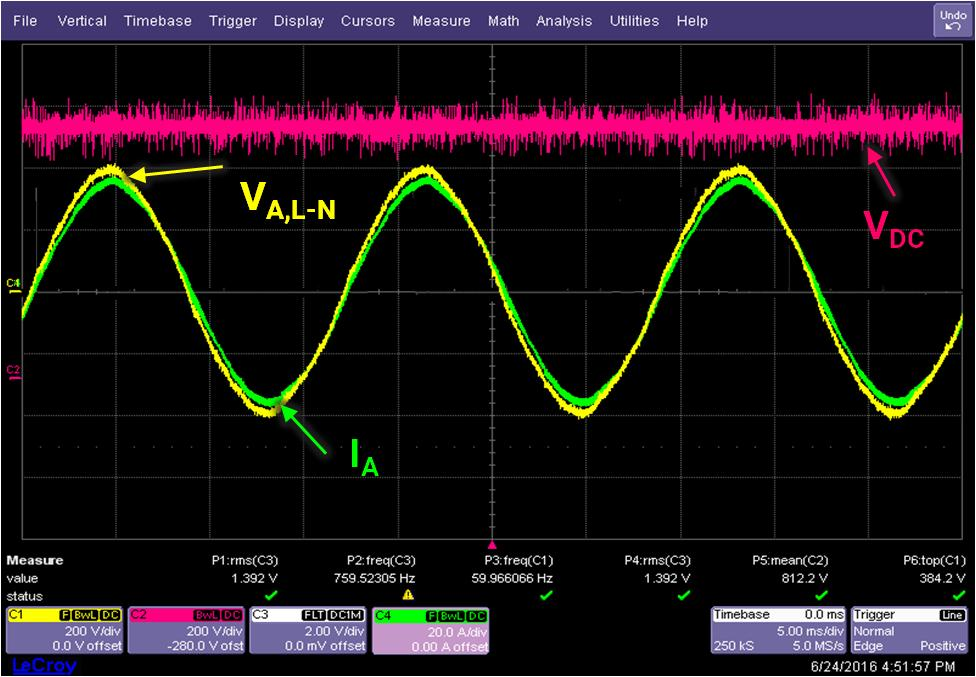Figure 9. Operating waveforms at full power. VGRID = 380V, VLINK = 800V, POUT = 20 kW, and FSW = 48 kHz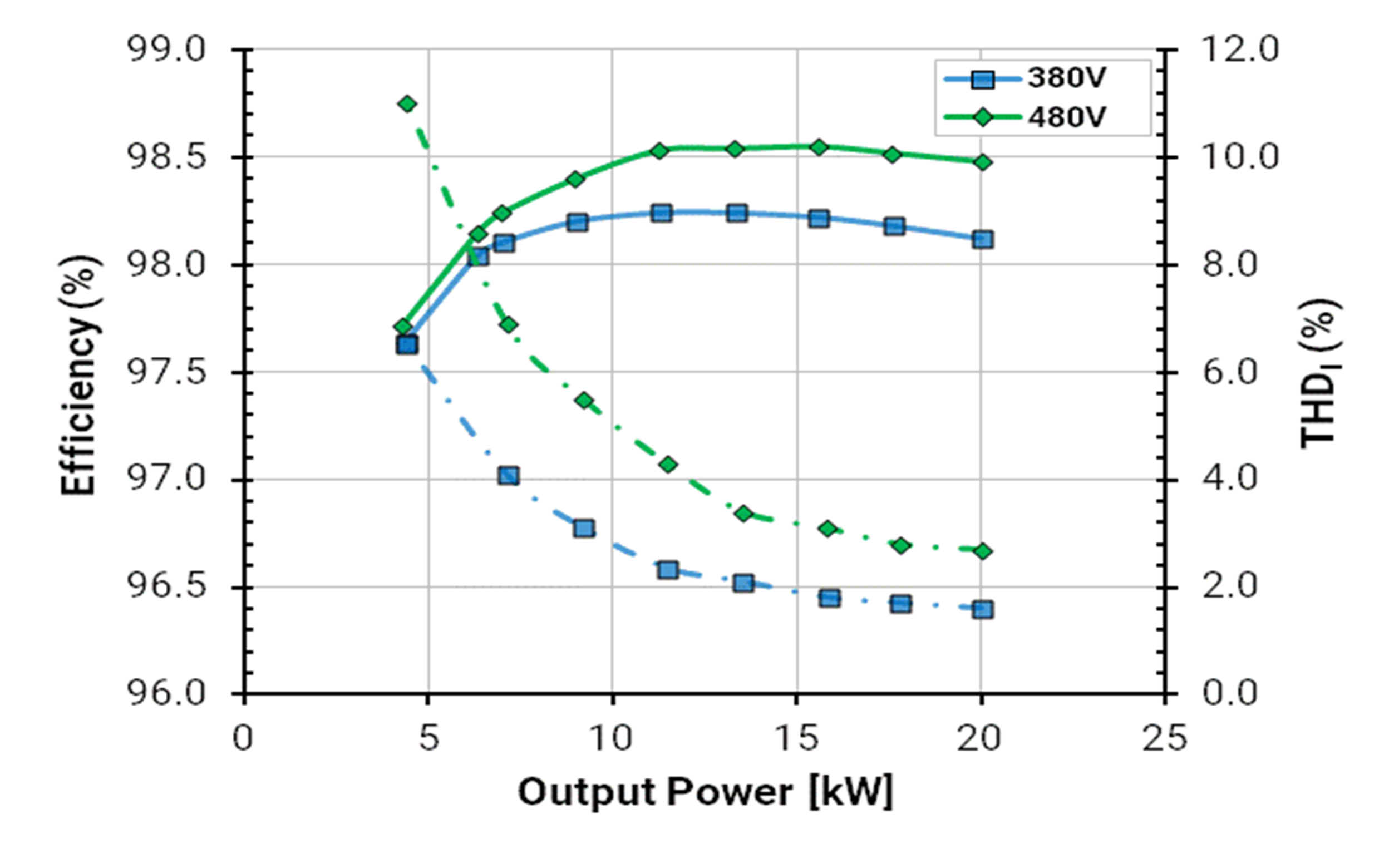Figure 10. Measued efficiency and THDI as a funtion of output power for various input voltages (FSW = 48 kHz and LPHASE = 400 µH).

Summary

This article demonstrates a design concept using commercially available SiC MOSFETs (C3M0065100K), which meets new market requirements for bidirectional functionality. A prototype 20-kW system in a simple two-level topology — switching at 48 kHz is shown. The prototype is tested to full power where measured results suggest a potential approximately 0.5-1.0 percent efficiency improvement over Si IGBT systems.

SiC MOSFETs used were TO-247-4L discrete packages with source Kelvin connection. The 1000 volt/65 mΩ SiC MOSFETs in TO-247-4L discrete packaging offer a cost-optimized solution, eliminating the need for anti-parallel SiC Schottky diodes. Any additional semiconductor cost is offset by the reduction in magnetic and cooling cost.